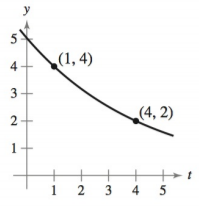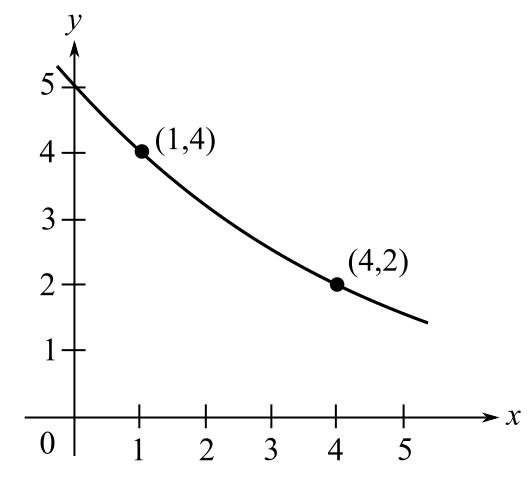Chapter 4.6, Problem 5E### Calculus: An Applied Approach (Min...

10th Edition
Ron Larson
ISBN: 9781305860919

#### Solutions

Chapter
Section### Calculus: An Applied Approach (Min...

10th Edition
Ron Larson
ISBN: 9781305860919
Textbook Problem
1 views

# Modeling Exponential Growth and Decay In Exercises 1-6, find the exponential function y = C e k t that passes through the two given points. y = C e k tTo determine

To calculate: The exponential function y=Cekt for the following graph:Explanation

Given Information:

The provided graph is:

Calculate:

Consider the following graph:

Observe that the exponential function curve passes through the points (1,4) and (4,2).

Hence the points (1,4) and (4,2) satisfy the equation of exponential function y=Cekt.

Substitute t=1,y=4 in the equation y=Cekt.

4=Cek(1)4=Cek

Substitute t=4,y=2 in the equation y=Cekt.

2=Cek(4)2=Ce4k

Now take the ratio of the equations Cek=4 and Ce4k=2,

Ce4kCek=24e4kek=12e4kk=12e3k=12

Take natural logarithm of both the sides.

ln(e3k)=ln(12)3k(lne)=ln(12)3k=ln(12)k=ln(12)3

Hence, the value of k is ln(12)3

### Still sussing out bartleby?

Check out a sample textbook solution.

See a sample solution

#### The Solution to Your Study Problems

Bartleby provides explanations to thousands of textbook problems written by our experts, many with advanced degrees!

Get Started

#### Find more solutions based on key concepts